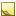﻿ Matrix4x4.ExtractYawPitchRoll Method# Matrix4x4AddLanguageSpecificTextSet("LST3722EB9C_0?cpp=::|nu=.");ExtractYawPitchRoll Method

Extract rotation angles from the rotation matrix.

Namespace:  Accord.Math
Assembly:  Accord.Math (in Accord.Math.dll) Version: 3.8.0Syntax
```public void ExtractYawPitchRoll(
out float yaw,
out float pitch,
out float roll
)```

#### Parameters

yaw
Type: SystemSingle
Extracted rotation angle around Y axis in radians.
pitch
Type: SystemSingle
Extracted rotation angle around X axis in radians.
roll
Type: SystemSingle
Extracted rotation angle around Z axis in radians.RemarksNote
The routine assumes roll-pitch-yaw rotation order when extracting rotation angle. Using extracted angles with the CreateFromYawPitchRoll(Single, Single, Single) should provide same rotation matrix.Note
The method assumes the provided matrix represent valid rotation matrix.

Sample usage:

```// assume we have a rotation matrix created like this
float yaw   = 10.0f / 180 * Math.PI;
float pitch = 30.0f / 180 * Math.PI;
float roll  = 45.0f / 180 * Math.PI;

Matrix4x4 rotationMatrix = Matrix3x3.CreateFromYawPitchRoll( yaw, pitch, roll );
// ...

// now somewhere in the code you may want to get rotation
// angles back from a matrix assuming same rotation order
float extractedYaw;
float extractedPitch;
float extractedRoll;

rotation.ExtractYawPitchRoll( out extractedYaw, out extractedPitch, out extractedRoll );```See Also